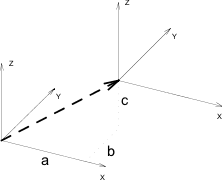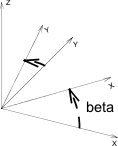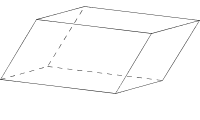## 3D Transformations

`ADDX dx`

`ADDY dy`

`ADDZ dz`

Moves the local coordinate system along the given axis by dx, dy or dz respectively.

`ADD dx, dy, dz`

Example:

`ADD a, b, c`It has only one entry in the stack, thus it can be deleted with DEL 1.

### MULX

`MULX mx`

### MULY

`MULY my`

### MULZ

`MULZ mz`

Scales the local coordinate system along the given axis. Negative mx, my, mz means simultaneous mirroring.

### MUL

`MUL mx, my, mz`

Replaces the sequence MULX mx: MULY my: MULZ mz. It has only one entry in the stack, thus it can be deleted with DEL 1.

### ROTX

`ROTX alphax`

### ROTY

`ROTY alphay`

### ROTZ

`ROTZ alphaz`

Rotates the local coordinate system around the given axis by alphax, alphay, alphaz degrees respectively, counterclockwise.

Example:

`ROTZ beta`### ROT

`ROT x, y, z, alpha`

Rotates the local coordinate system around the axis defined by the vector (x, y, z) by alpha degrees, counterclockwise.
It has only one entry in the stack, thus it can be deleted with DEL 1.

### XFORM

```XFORM newx_x, newy_x, newz_x, offset_x,
newx_y, newy_y, newz_y, offset_y,
newx_z, newy_z, newz_z, offset_z```

Defines a complete transformation matrix. It is mainly used in
automatic GDL code generation. It has only one entry in the stack.

x’ = newx_x * x + newy_x * y + newz_x * z + offset_x

y’ = newx_y * x + newy_y * y + newz_y * z + offset_y

z’ = newx_z * x + newy_z * y + newz_z * z + offset_z

Example code:

 ```A = 60 B = 30 XFORM 2, COS(A), COS(B)*0.6, 0, 0, SIN(A), SIN(B)*0.6, 0, 0, 0, 1, 0 BLOCK 1, 1, 1```

… and it’s 3D view: